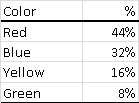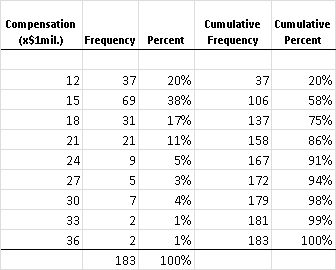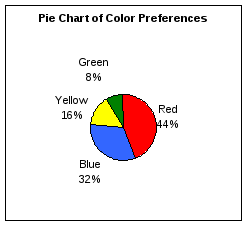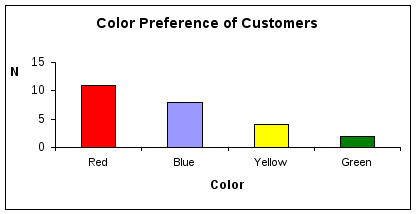# Describing and Interpreting Data

 Page 1/3 Date 16.06.2018 Size 195 Kb.
1   2   3
Overview

Describing and Interpreting Data
The manner in which you analyze data depends on the type of data/variables that you are evaluating. There are several different classifications that are used in classifying data.
Variable

• A variable is an item of data

• Examples of variables include quantities such as: gender, investment type, test scores, and weight. The values of these quantities vary from one observation to another.

Types/Classifications of Variables

• Qualitative: Non-numerical quality

• Quantitative: Numerical

Qualitative Data

• This data describes the quality of something in a non-numerical format.

• Counts can be applied to qualitative data, but you cannot order or measure this type of variable. Examples are gender, marital status, geographical region of an organization, job title….

• Qualitative data is usually treated as Categorical Data.

With categorical data, the observations can be sorted according into non-overlapping categories or by characteristics.

• For example, shirts can be sorted according to color; the characteristic 'color' can have non-overlapping categories: white, black, red, etc. People can be sorted by gender with categories male and female.

• Categories should be chosen carefully since a bad choice can prejudice the outcome. Every value of a data set should belong to one and only one category.

• Measurement Scale

• Nominal: classifies with no ranking (e.g. color, investment type...)

• Ordinal: classifies with ranking (e.g. product satisfaction, grades…)

• Analyze qualitative data using:

• Frequency tables, Contingency tables (for 2 variables)

• Modes - most frequently occurring

• Graphs: Bar Charts, Pie Charts, Pareto Charts

Quantitative Data

• Quantitative or numerical data arise when the observations are frequencies or measurements.

• Discrete Data

• The data are said to be discrete if the measurements are integers (e.g. number of employees of a company, number of incorrect answers on a test, number of participants in a program…)

• Continuous Data

• The data are said to be continuous if the measurements can take on any value, usually within some range (e.g. weight). Age and income are continuous quantitative variables. For continuous variables, arithmetic operations such as differences and averages make sense.

• Analysis can take almost any form:

• Create groups or categories and generate frequency tables.

• Effective graphs include: Histograms, Stem-and-Leaf plots, Dot Plots, Box plots, and XY Scatter Plots (2 variables).

• All descriptive statistics can be applied.

• Measurement Scale

• Interval: ordered and difference between variables is meaningful (e.g. standardized scores...)

• Ratio: ordered and difference between variables is meaningful, true 0 in measuring

Note: Some “quantitative” variables can be treated only as ranks; they have a natural order, but these values are not strictly measured (ordinal data). Examples are: 1) age group (taking the values child, teen, adult, senior), and 2) Likert Scale data (responses such as strongly agree, agree, neutral, disagree, strongly disagree). For these variables, the distinction between adjacent points on the scale is not necessarily the same, and the ratio of values is not meaningful.

• Analyze using:

• Frequency tables

• Mode, Median, Quartiles

• Graphs: Bar Charts, Dot Plots, Pie Charts, and Line Charts (2 variables)

Tables
Frequency Table/Frequency Distribution

A frequency or relative frequency table is used to summarize categorical, nominal, and ordinal data. It is also be used to summarize continuous data when the data set has been divided into meaningful groups.

Count the number of observations that fall into each category. The number associated with each category is called the frequencyand the collection of frequencies over all categories gives the frequency distribution of that variable. Generally, a frequency distribution has 5 to 15 classes.

• It presents data in a useful form and allows for a visual interpretation.

• It enables analysis of the data set including where the data are concentrated / clustered, the range of values, and observation of extreme values,

Frequency Table for Qualitative Data
Color Preferences of CustomersFrequency Distribution for Quanitative Data

 Table 1 Frequency Distribution of Time (min)

Note Table1

There are 8 classes. The frequency of the first class is 1; i.e. there is 1 value within the class; the class has a midpoint of 110.

 Time Count 110 1 115 2 120 4 125 3 130 5 135 3 140 4 145 2 150 1

The relative frequency is a number which describes the proportion of observations falling in a given category. Instead of counts, we report relative frequencies or percentages.

CEO Compensation (x\$1 mil.)Contingency Table

A contingency table cross tabulates data using two or more categorical variables to allow for analysis of relationships between the variables.

Table C: Employee Time at Company (. 3 yrs.) by Prior Related Experience Rating

 Count of Prior Related Exp. Rating Stayed3Yrs Prior Related Exp. Rating No Yes Grand Total Very Good 8 6 14 Good 16 15 31 Fair 8 9 17 Minimal 2 2 4 Grand Total 34 32 66

Graphs

Note Excel will create any graph that you specify, even if the graph that you select is not appropriate for the data. Remember - consider the type of data that you have before selecting your graph.

Graphs Used for Categorical/ Qualitative Data
Pie Charts

A circle is divided proportionately and shows what percentage of the whole falls into each category. The size of each slice of the pie varies according to the percentage in each category.

• These charts are simple to understand.

• They convey information regarding the relative size of groups more readily than does a table.

Bar Charts

Bar charts also show percentages in various categories and allow comparison between categories.

• The vertical scale is frequencies, relative frequencies, or percentages.

• The horizontal scale shows categories.

• Consider the following in constructing bar charts.

• all boxes should have the same width

• leave gaps between the boxes (because there is no connection between them)

• boxes can be in any order.

• Bar charts can be used to represent two categorical variables simultaneouslyAs presented above, the bar chart is also called a Pareto chart because the vertical bars are plotted in descending order by frequency (i.e. red is the most frequent selection …green occurs the least frequent.) They are used to separate the “vital few” from the “trivial many.